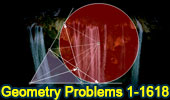# Geometry Problem 453: Intersecting Circles, Tangent, Circular Segment, Congruence, Area

 The figure shows the intersecting circles O and O' at A and B. Line CO is tangent to circle O' at O, lines CDA and CO' are chords of circle O. Prove that the circular segments S and S' are congruent. A circular segment is an area of a circle enclosed between a chord and the arc whose endpoints equal the chord's.More Problems: Proposed Problem 454. Triangle, Incircle, Inradius, Tangent, Circle, Radius. Proposed Problem 452. Triangle, Circle, Tangent, Proportional Segments. Proposed Problem 451. Triangle, Median, Congruence, Proportional Segments. Proposed Problem 450. Triangle, Median, Cevian, Congruence, Proportional Segments.Home | Search | Geometry | Problems | All Problems | Visual Index | 451-460 | Circular Segment | Email | Post a comment or solution | By Antonio Gutierrez